This mathematics ClipArt gallery offers 13 images of the graphs of the six basic trigonometric functions: sine, cosine, tangent, cotangent, secant, and cosecant.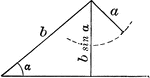### Ambiguous Case

Illustration of one possible outcome (no triangle occurs) when discussing the ambiguous case using the…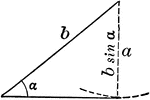### Ambiguous Case

Illustration of one possible outcome (1 triangle occurs) when discussing the ambiguous case using the…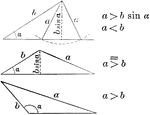### Ambiguous Case

Illustration of one possible outcome (2 triangles occur) when discussing the ambiguous case using the…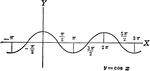### Cosine Curve y=cos x

Cosine curve plotted from negative pi to 2 pi. Graph of y=cos x.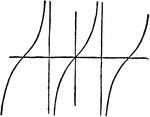### Tangent Curve

Illustration showing a tangent curve.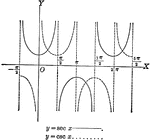### Secant and Cosecant Curves, y=sec x and y=csc x

Secant and Cosecant curves plotted from negative pi to 2 pi. Graph of y=sec x and y=csc x.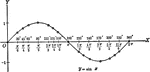### Sine Curve y=sin x

Sine curve plotted from 0 to 2 pi. Graph of y=sin x.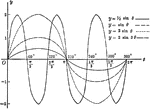### Sine Curves y= sin Ǝ, y= 1/2 sin Ǝ, y=2 sin Ǝ, y= 2 sin 3Ǝ

Sine curves of varying frequency and amplitude plotted from 0 to 2 pi. Graph of y= sin θ, y= 1/2…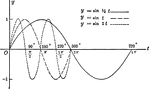### Sine Curves y= sin t, y= r sin1/2t, y= r sin 2t

Sine curves of varying frequency plotted from 0 to 2 pi. Graph of y= sin t, y= r sin1/2t, y=r sin 2t.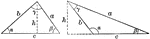### Law of Sines

Illustration two types of triangles that can be used to model the law of sines. "In a plane triangle…### Sinusoid or Sine Wave

"The curve of sines, in which the abscissas are proportional to an angle, and the ordinates to its sine."…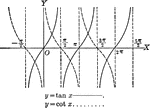### Tangent and Cotangent Curves, y=tan x and y=cot x

Tangent and Cotangent curves plotted from negative pi to 2 pi. Graph of y=tan x and y=cot x.